# 5.5 Zeros of polynomial functions  (Page 8/14)

 Page 8 / 14

$\left({x}^{4}-1\right)÷\left(x-4\right)$

$255$

$\left(3{x}^{3}+4{x}^{2}-8x+2\right)÷\left(x-3\right)$

$\left(4{x}^{3}+5{x}^{2}-2x+7\right)÷\left(x+2\right)$

$-1$

For the following exercises, use the Factor Theorem to find all real zeros for the given polynomial function and one factor.

$f\left(x\right)=2{x}^{3}+3{x}^{2}+x+6;\text{\hspace{0.17em}}\text{\hspace{0.17em}}\text{\hspace{0.17em}}\text{\hspace{0.17em}}x+2$

$-2$

$f\left(x\right)=-5{x}^{3}+16{x}^{2}-9;\text{\hspace{0.17em}}\text{\hspace{0.17em}}\text{\hspace{0.17em}}\text{\hspace{0.17em}}x-3$

${x}^{3}+3{x}^{2}+4x+12;\text{\hspace{0.17em}}x+3$

$-3$

$4{x}^{3}-7x+3;\text{\hspace{0.17em}}x-1$

$2{x}^{3}+5{x}^{2}-12x-30,\text{​}\text{\hspace{0.17em}}2x+5$

For the following exercises, use the Rational Zero Theorem to find all real zeros.

${x}^{3}-3{x}^{2}-10x+24=0$

$2{x}^{3}+7{x}^{2}-10x-24=0$

${x}^{3}+2{x}^{2}-9x-18=0$

${x}^{3}+5{x}^{2}-16x-80=0$

${x}^{3}-3{x}^{2}-25x+75=0$

$2{x}^{3}-3{x}^{2}-32x-15=0$

$2{x}^{3}+{x}^{2}-7x-6=0$

$2{x}^{3}-3{x}^{2}-x+1=0$

$3{x}^{3}-{x}^{2}-11x-6=0$

$2{x}^{3}-5{x}^{2}+9x-9=0$

$\frac{3}{2}$

$2{x}^{3}-3{x}^{2}+4x+3=0$

${x}^{4}-2{x}^{3}-7{x}^{2}+8x+12=0$

${x}^{4}+2{x}^{3}-9{x}^{2}-2x+8=0$

$4{x}^{4}+4{x}^{3}-25{x}^{2}-x+6=0$

$2{x}^{4}-3{x}^{3}-15{x}^{2}+32x-12=0$

${x}^{4}+2{x}^{3}-4{x}^{2}-10x-5=0$

$4{x}^{3}-3x+1=0$

$8{x}^{{}^{4}}+26{x}^{3}+39{x}^{2}+26x+6$

For the following exercises, find all complex solutions (real and non-real).

${x}^{3}+{x}^{2}+x+1=0$

${x}^{3}-8{x}^{2}+25x-26=0$

${x}^{3}+13{x}^{2}+57x+85=0$

$3{x}^{3}-4{x}^{2}+11x+10=0$

${x}^{4}+2{x}^{3}+22{x}^{2}+50x-75=0$

$2{x}^{3}-3{x}^{2}+32x+17=0$

## Graphical

For the following exercises, use Descartes’ Rule to determine the possible number of positive and negative solutions. Confirm with the given graph.

$f\left(x\right)={x}^{3}-1$

$f\left(x\right)={x}^{4}-{x}^{2}-1$

1 positive, 1 negative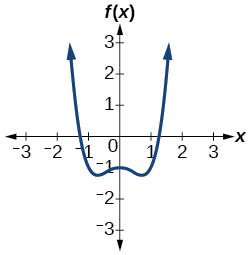$f\left(x\right)={x}^{3}-2{x}^{2}-5x+6$

$f\left(x\right)={x}^{3}-2{x}^{2}+x-1$

3 or 1 positive, 0 negative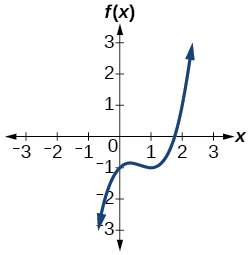$f\left(x\right)={x}^{4}+2{x}^{3}-12{x}^{2}+14x-5$

$f\left(x\right)=2{x}^{3}+37{x}^{2}+200x+300$

0 positive, 3 or 1 negative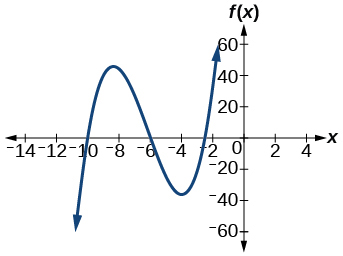$f\left(x\right)={x}^{3}-2{x}^{2}-16x+32$

$f\left(x\right)=2{x}^{4}-5{x}^{3}-5{x}^{2}+5x+3$

2 or 0 positive, 2 or 0 negative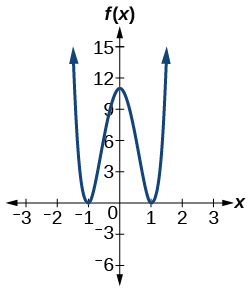$f\left(x\right)=2{x}^{4}-5{x}^{3}-14{x}^{2}+20x+8$

$f\left(x\right)=10{x}^{4}-21{x}^{2}+11$

2 or 0 positive, 2 or 0 negative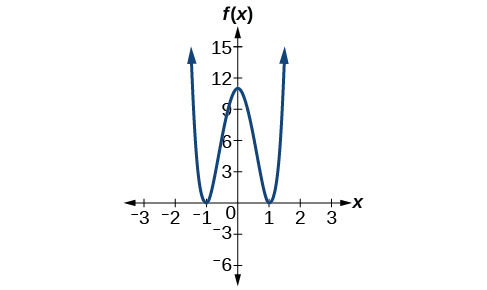## Numeric

For the following exercises, list all possible rational zeros for the functions.

$f\left(x\right)={x}^{4}+3{x}^{3}-4x+4$

$f\left(x\right)=2{x}^{{}^{3}}+3{x}^{2}-8x+5$

$f\left(x\right)=3{x}^{{}^{3}}+5{x}^{2}-5x+4$

$f\left(x\right)=6{x}^{4}-10{x}^{2}+13x+1$

$f\left(x\right)=4{x}^{5}-10{x}^{4}+8{x}^{3}+{x}^{2}-8$

## Technology

For the following exercises, use your calculator to graph the polynomial function. Based on the graph, find the rational zeros. All real solutions are rational.

$f\left(x\right)=6{x}^{3}-7{x}^{2}+1$

$f\left(x\right)=4{x}^{3}-4{x}^{2}-13x-5$

$f\left(x\right)=8{x}^{3}-6{x}^{2}-23x+6$

$f\left(x\right)=12{x}^{4}+55{x}^{3}+12{x}^{2}-117x+54$

$f\left(x\right)=16{x}^{4}-24{x}^{3}+{x}^{2}-15x+25$

$\frac{5}{4}$

## Extensions

For the following exercises, construct a polynomial function of least degree possible using the given information.

Real roots: –1, 1, 3 and $\text{\hspace{0.17em}}\left(2,f\left(2\right)\right)=\left(2,4\right)$

Real roots: –1 (with multiplicity 2 and 1) and $\text{\hspace{0.17em}}\left(2,f\left(2\right)\right)=\left(2,4\right)$

$f\left(x\right)=\frac{4}{9}\left({x}^{3}+{x}^{2}-x-1\right)$

Real roots: –2, $\text{\hspace{0.17em}}\frac{1}{2}\text{\hspace{0.17em}}$ (with multiplicity 2) and $\text{\hspace{0.17em}}\left(-3,f\left(-3\right)\right)=\left(-3,5\right)$

Real roots: $\text{\hspace{0.17em}}-\frac{1}{2}$ , 0, $\text{\hspace{0.17em}}\frac{1}{2}\text{\hspace{0.17em}}$ and $\text{\hspace{0.17em}}\left(-2,f\left(-2\right)\right)=\left(-2,6\right)$

$f\left(x\right)=-\frac{1}{5}\left(4{x}^{3}-x\right)$

Real roots: –4, –1, 1, 4 and $\text{\hspace{0.17em}}\left(-2,f\left(-2\right)\right)=\left(-2,10\right)$

## Real-world applications

For the following exercises, find the dimensions of the box described.

The length is twice as long as the width. The height is 2 inches greater than the width. The volume is 192 cubic inches.

8 by 4 by 6 inches

The length, width, and height are consecutive whole numbers. The volume is 120 cubic inches.

The length is one inch more than the width, which is one inch more than the height. The volume is 86.625 cubic inches.

5.5 by 4.5 by 3.5 inches

The length is three times the height and the height is one inch less than the width. The volume is 108 cubic inches.

The length is 3 inches more than the width. The width is 2 inches more than the height. The volume is 120 cubic inches.

8 by 5 by 3 inches

For the following exercises, find the dimensions of the right circular cylinder described.

The radius is 3 inches more than the height. The volume is $\text{\hspace{0.17em}}16\pi \text{\hspace{0.17em}}$ cubic meters.

The height is one less than one half the radius. The volume is $\text{\hspace{0.17em}}72\pi \text{\hspace{0.17em}}$ cubic meters.

Radius = 6 meters, Height = 2 meters

The radius and height differ by one meter. The radius is larger and the volume is $\text{\hspace{0.17em}}48\pi \text{\hspace{0.17em}}$ cubic meters.

The radius and height differ by two meters. The height is greater and the volume is $\text{\hspace{0.17em}}28.125\pi \text{\hspace{0.17em}}$ cubic meters.

Radius = 2.5 meters, Height = 4.5 meters

The radius is $\text{\hspace{0.17em}}\frac{1}{3}\text{\hspace{0.17em}}$ meter greater than the height. The volume is $\text{\hspace{0.17em}}\frac{98}{9}\pi \text{\hspace{0.17em}}$ cubic meters.

#### Questions & Answers

write down the polynomial function with root 1/3,2,-3 with solution
if A and B are subspaces of V prove that (A+B)/B=A/(A-B)
write down the value of each of the following in surd form a)cos(-65°) b)sin(-180°)c)tan(225°)d)tan(135°)
Prove that (sinA/1-cosA - 1-cosA/sinA) (cosA/1-sinA - 1-sinA/cosA) = 4
what is the answer to dividing negative index
In a triangle ABC prove that. (b+c)cosA+(c+a)cosB+(a+b)cisC=a+b+c.
give me the waec 2019 questions
the polar co-ordinate of the point (-1, -1)
prove the identites sin x ( 1+ tan x )+ cos x ( 1+ cot x )= sec x + cosec x
tanh`(x-iy) =A+iB, find A and B
B=Ai-itan(hx-hiy)
Rukmini
what is the addition of 101011 with 101010
If those numbers are binary, it's 1010101. If they are base 10, it's 202021.
Jack
extra power 4 minus 5 x cube + 7 x square minus 5 x + 1 equal to zero
the gradient function of a curve is 2x+4 and the curve passes through point (1,4) find the equation of the curve
1+cos²A/cos²A=2cosec²A-1
test for convergence the series 1+x/2+2!/9x3ByBy